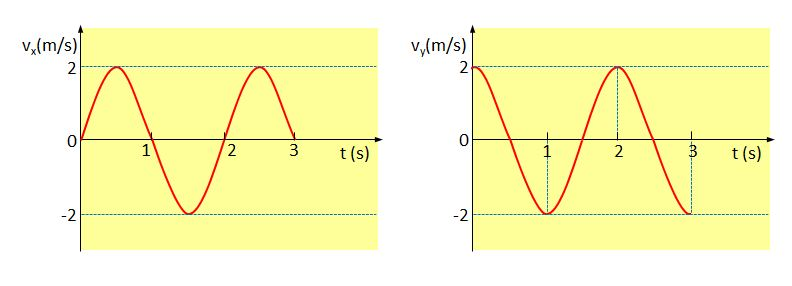# Velocity components of a circular motionThe above are the velocity component graphs of a particular object in uniform circular motion on the $xy$-plane, as a function of time. Which of the following statements is correct about the motion of this object?

a) The speed of the object is constant at $2$ m/s.

b) The magnitude of the acceleration of the object is constant.

c) The angular speed of the object is $2\pi$ rad/s.

×